# Garden

Garden with an area of 800 square meters rained 3 mm of water. How many 10-liter cans of water is needed to same irrigation?

Correct result:

n =  240

#### Solution: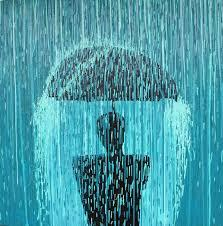We would be pleased if you find an error in the word problem, spelling mistakes, or inaccuracies and send it to us. Thank you!Tips to related online calculators
Do you want to convert length units?
Do you know the volume and unit volume, and want to convert volume units?

#### You need to know the following knowledge to solve this word math problem:

We encourage you to watch this tutorial video on this math problem:

## Next similar math problems:

• Rainwater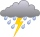6 mm of water rained on the garden with an area of 25 acres. How many 12 liter cans of water would we water this garden just as much?
• Rain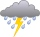Garden shape of a rectangle measuring 15 m and 20 m rained water up to 3 mm. How many liters of water rained on the garden?
• Water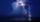On the lawn which has area 914 m2 rained 3 mm of water. How many liters of water rained?
• MilimetersThe pool is 6 meters long, 3 meters wide and the water in it is filled with water to a height 1.7 m. When John jumped into it and completely submerged, the level has risen by 5.4 mm. How much weight John when we know that one liter of the human body weigh
• Annual rainfallThe average annual rainfall is 686 mm. How many liters will fall on the 1-hectare field?
• RainfallHow many liters of water did fell in a 32m long and 8m wide garden, if 8mm of rain fell?
• RainfallAnnual rainfall in our country is an average of 797 mm. How many m3 of water rains on average per hectare?
• FlowerbedThe flowerbed has a length 3500mm and a width 1400mm. How many foil is needed to covers the flowerbed? How many m2 of foil was consumed for its production (add 10% of the material to the joint and waste)? How many liters of air is inside the enclosure? (F
• TetrapackHow high should be the milk box in the shape of a prism with base dimensions 8 cm and 8.8 cm if its volume is 1 liter?
• PoolMr. Peter builds a pool shape of a four-sided prism with a rhombus base in the garden. Base edge length is 8 m, the distance of the opposite walls of the pool is 7 m. The estimated depth is 144 cm. How many hectoliters of water consume Mr. Peter to fill t
• Water tankWater tank shape of cuboid has dimensions of the bottom 7.5 meters and 3 meters. How high will reach the water in the tank will flow 10 liters of water per second and the inflow will be open 5/6 hour? (Calculate to one decimal place and the results give i
• RainfallA rectangular garden of 25m in length and width 20m in width fall 4mm of water. Express by a fraction in basic form what part of the 60-hectolitre tank we would fill with this water.
• The potThe pot is in 1/3 filled with water. Bottom of the pot has an area of ​​329 cm2. How many centimeters rises water level in the pot after add 1.2 liters of water?
• Water tank300hl of water was filled into the tank 12 m long and 6 m wide. How high does it reach?
• Three-quarters of its volume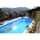The pool has a block shape with a length of 8m, a width of 5.3m and a depth of 1.5m. How many hl of water is in it if it is filled to three-quarters of its volume?
• Water poolWhat water level is in the pool shaped cuboid with bottom dimensions of 25 m and 10 meters, when is 3750hl water in the pool.
• Four prismsQuestion No. 1: The prism has the dimensions a = 2.5 cm, b = 100 mm, c = 12 cm. What is its volume? a) 3000 cm2 b) 300 cm2 c) 3000 cm3 d) 300 cm3 Question No.2: The base of the prism is a rhombus with a side length of 30 cm and a height of 27 cm. The heig